# 探索标准库的交易策略类 - 自定义策略

19 三月 2014, 05:57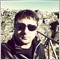0
1 890### MQL5 向导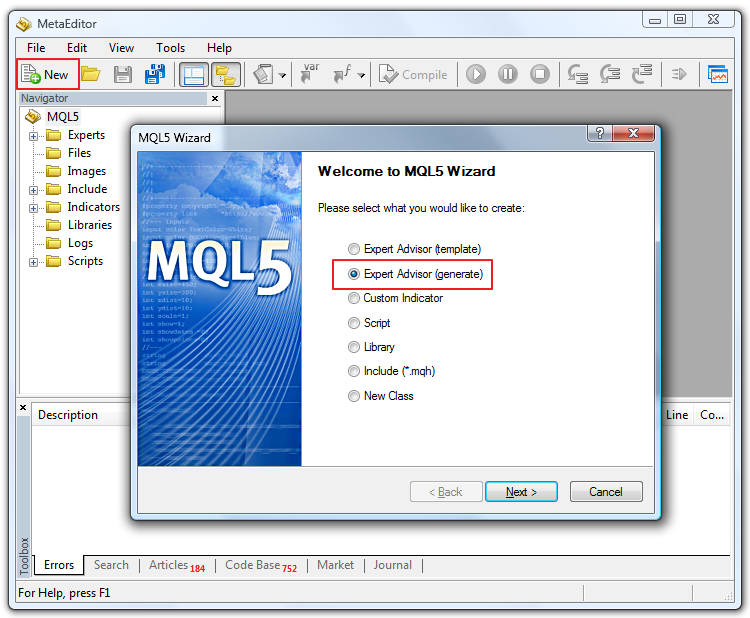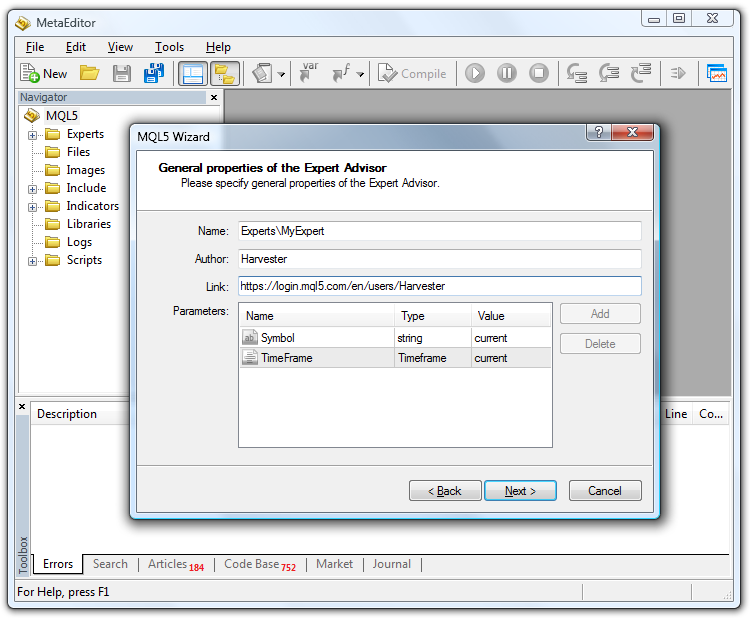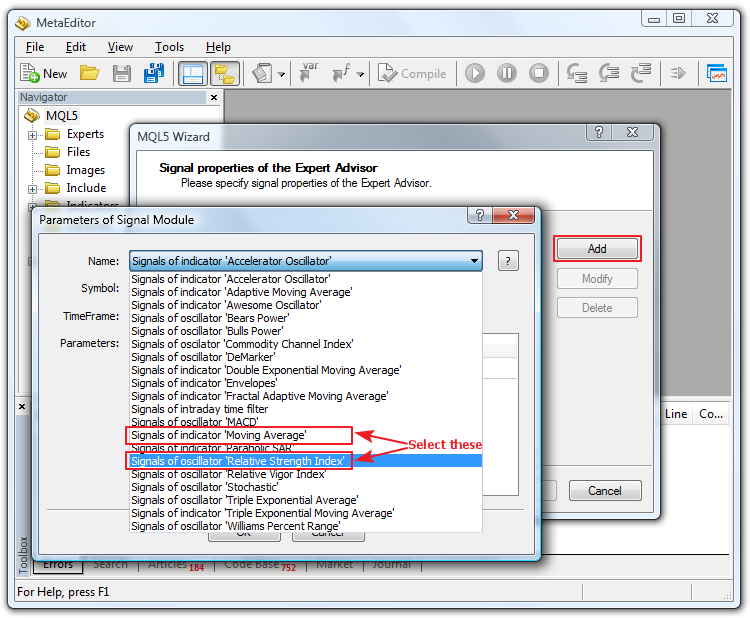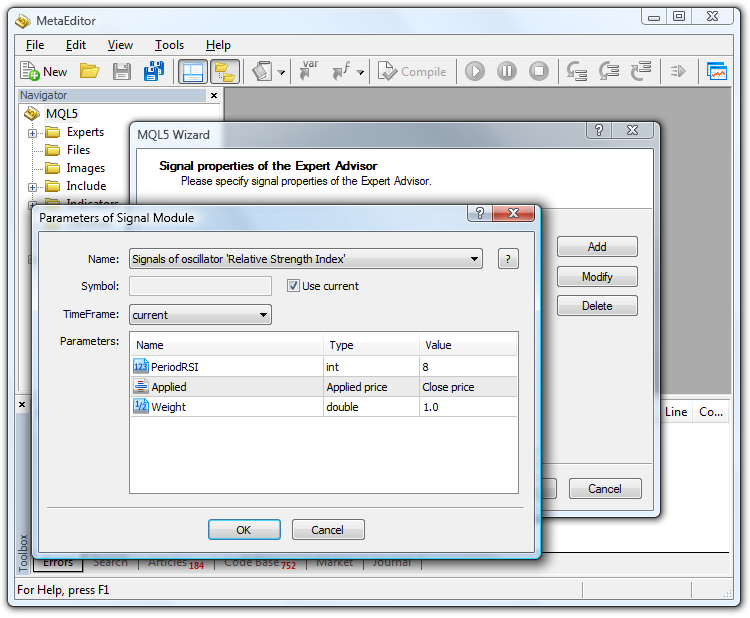### 分析生成的代码

```//+------------------------------------------------------------------+
//|                                                     MyExpert.mq5 |
//|                                                        Harvester |
//|                        https://www.mql5.com/en/users/Harvester |
//+------------------------------------------------------------------+
#property version   "1.00"
//+------------------------------------------------------------------+
//| Include                                                          |
//+------------------------------------------------------------------+
#include <Expert\Expert.mqh>
//--- available signals
#include <Expert\Signal\SignalRSI.mqh>
#include <Expert\Signal\SignalMA.mqh>
//--- available trailing
#include <Expert\Trailing\TrailingNone.mqh>
//--- available money management
#include <Expert\Money\MoneyFixedLot.mqh>```

• Expert.mqh
• SignalRSI.mq
• SignalMA.mqh

```//--- Creating filter CSignalRSI
CSignalRSI *filter0=new CSignalRSI;
if(filter0==NULL)
{
//--- failed
printf(__FUNCTION__+": error creating filter0");
ExtExpert.Deinit();
return(-3);
}

CSignalRSI 表示类信号 RSI。此类用于调用 RSI 指标，并向其应用一些条件以通过使用向导的模式逻辑创建买入或卖出信号。RSI 是我们的第一个过滤器（过滤器编号 0）。

```//--- Tuning of all necessary indicators
if(!ExtExpert.InitIndicators())
{
//--- failed
printf(__FUNCTION__+": error initializing indicators");
ExtExpert.Deinit();
return(-10);
}
//--- ok
return(0);
}```

```//+------------------------------------------------------------------+
//| Deinitialization function of the expert                          |
//+------------------------------------------------------------------+
void OnDeinit(const int reason)
{
ExtExpert.Deinit();
}
//+------------------------------------------------------------------+
//| "Tick" event handler function                                    |
//+------------------------------------------------------------------+
void OnTick()
{
ExtExpert.OnTick();
}
//+------------------------------------------------------------------+
//| "Trade" event handler function                                   |
//+------------------------------------------------------------------+
{
}
//+------------------------------------------------------------------+
//| "Timer" event handler function                                   |
//+------------------------------------------------------------------+
void OnTimer()
{
ExtExpert.OnTimer();
}
//+------------------------------------------------------------------+```

```//--- Creating filter CSignalCCIxx
CSignalCCIxx *filter2=new CSignalCCIxx;
if(filter2==NULL)
{
//--- failed
printf(__FUNCTION__+": error creating filter2");
ExtExpert.Deinit();
return(-4);
}
//--- Set filter parameters
filter2.PeriodCCIxx(Signal_CCIxx_PeriodCCI);
filter2.Applied(Signal_CCIxx_Applied);
filter2.Weight(Signal_CCIxx_Weight);```

• #include "ExpertBase.mqh"
• #include "ExpertSignal.mqh"
• #include "ExpertMoney.mqh"
• #include "ExpertTrailing.mqh"

```//+------------------------------------------------------------------+
//| Include                                                          |
//+------------------------------------------------------------------+
#include <Expert\Expert.mqh>
//--- available signals
#include <Expert\Signal\SignalRSI.mqh>
#include <Expert\Signal\SignalMA.mqh>

#include <Expert\Signal\SignalCCIxx.mqh>   // This is our own 'custom' indicator for custom Signal management of the EA```

SignalCCIxx.mqh 文件应位于 \MQL5\Include\Expert\Signal\ 文件夹中，并且与向导生成 EA 的可集成性相一致，就像标准库的其他 #include 交易类一样 - 信号文件已经在此文件夹中（SignalRSI.mqh 和 SignalMA.mqh）。

### 自定义策略

EA 将只是交易并且是完美地交易，至少对于那些希望参赛但不会编程也不想在工作服务（对于锦标赛参赛者而言是个不错的选择）中订购 EA 的参赛者而言是如此。有大量的输入参数可供指定，以使您的自动交易尽可能贴近于您脑海中的策略构思。

```//+------------------------------------------------------------------+
//| Inputs                                                           |
//+------------------------------------------------------------------+
//--- inputs for expert
input string             Expert_Title         ="MyExpert";  // Document name
ulong                    Expert_MagicNumber   =26287;       //
bool                     Expert_EveryTick     =false;       //
//--- inputs for main signal
input int                Signal_ThresholdOpen =40;          // Signal threshold value to open [0...100]
input int                Signal_ThresholdClose=60;          // Signal threshold value to close [0...100]
input double             Signal_PriceLevel    =0.0;         // Price level to execute a deal
input double             Signal_StopLevel     =50.0;        // Stop Loss level (in points)
input double             Signal_TakeLevel     =50.0;        // Take Profit level (in points)
input int                Signal_Expiration    =4;           // Expiration of pending orders (in bars)
input int                Signal_RSI_PeriodRSI =8;           // Relative Strength Index(8,...) Period of calculation
input ENUM_APPLIED_PRICE Signal_RSI_Applied   =PRICE_CLOSE; // Relative Strength Index(8,...) Prices series
input double             Signal_RSI_Weight    =0.7;         // Relative Strength Index(8,...) Weight [0...1.0]
input int                Signal_MA_PeriodMA   =90;          // Moving Average(12,0,...) Period of averaging
input int                Signal_MA_Shift      =0;           // Moving Average(12,0,...) Time shift
input ENUM_MA_METHOD     Signal_MA_Method     =MODE_SMA;    // Moving Average(12,0,...) Method of averaging
input ENUM_APPLIED_PRICE Signal_MA_Applied    =PRICE_CLOSE; // Moving Average(12,0,...) Prices series
input double             Signal_MA_Weight     =0.6;         // Moving Average(12,0,...) Weight [0...1.0]

input int                Signal_CCIxx_PeriodCCI =8;            // Commodity Channel Index(8,...) Period of calculation
input ENUM_APPLIED_PRICE Signal_CCIxx_Applied   =PRICE_CLOSE;  // Commodity Channel Index(8,...) Prices series
input double             Signal_CCIxx_Weight    =0.8;          // Commodity Channel Index(8,...) Weight [0...1.0]```

```class CSignalCCI : public CExpertSignal
{
protected:
CiCCI             m_cci;            // object-oscillator
int               m_periodCCI;      // the "period of calculation" parameter of the oscillator
ENUM_APPLIED_PRICE m_applied;       // the "prices series" parameter of the oscillator
//--- "weights" of market models (0-100)
int               m_pattern_0;      // model 0 "the oscillator has required direction"
int               m_pattern_1;      // model 1 "reverse behind the level of overbuying/overselling"
int               m_pattern_2;      // model 2 "divergence of the oscillator and price"
int               m_pattern_3;      // model 3 "double divergence of the oscillator and price"
//--- variables
double            m_extr_osc;   // array of values of extremums of the oscillator
double            m_extr_pr;    // array of values of the corresponding extremums of price
int               m_extr_pos;   // array of shifts of extremums (in bars)
uint              m_extr_map;       // resulting bit-map of ratio of extremums of the oscillator and the price```

```//--- "weights" of market models (0-100)
int               m_pattern_0;      // model 0 "the oscillator has required direction"
int               m_pattern_1;      // model 1 "reverse behind the level of overbuying/overselling"
int               m_pattern_2;      // model 2 "divergence of the oscillator and price"
int               m_pattern_3;      // model 3 "double divergence of the oscillator and price"

int               m_pattern_4;      // model 4 "our own first new pattern: values cross the zero"
int               m_pattern_5;      // model 5 "our own second new pattern: values bounce around the zero"```

```//+------------------------------------------------------------------+
//| Class CSignalCCIxx.                                              |
//| Purpose: Class of generator of trade signals based on            |
//|          the 'Commodity Channel Index' oscillator.               |
//| Is derived from the CExpertSignal class.                         |
//+------------------------------------------------------------------+
class CSignalCCIxx : public CExpertSignal
{
protected:
CiCCIxx             m_CCIxx;            // object-oscillator```

```protected:
CiCCIxx             m_CCIxx;            // object-oscillator```

```protected:
CiCCI             m_CCIxx;            // object-oscillator```

```//+------------------------------------------------------------------+
//| Constructor CSignalCCIxx.                                        |
//| INPUT:  no.                                                      |
//| OUTPUT: no.                                                      |
//| REMARK: no.                                                      |
//+------------------------------------------------------------------+
void CSignalCCIxx::CSignalCCIxx()
{
//--- initialization of protected data
m_used_series=USE_SERIES_HIGH+USE_SERIES_LOW;
//--- setting default values for the oscillator parameters
m_periodCCIxx  =14;
//--- setting default "weights" of the market models
m_pattern_0  =90;         // model 0 "the oscillator has required direction"
m_pattern_1  =60;         // model 1 "reverse behind the level of overbuying/overselling"
m_pattern_2  =100;        // model 2 "divergence of the oscillator and price"
m_pattern_3  =50;         // model 3 "double divergence of the oscillator and price"
m_pattern_4  =90;         // model 4 "our own first new pattern: "
m_pattern_5  =90;         // model 5 "our own second new pattern:
}```

### 第一个模式

• 图 5 显示对应于柱 1（当前柱的前一个柱）的 CCI 值。其值 45.16 因此 > 0。
• 图 6 显示对应于柱 2（当前柱的前两个柱）的 CCI 值。其值 -53.92 因此 < 0。
• 在 2 个柱内，CCI 指标的零线（值 0.00）被自下而上穿越。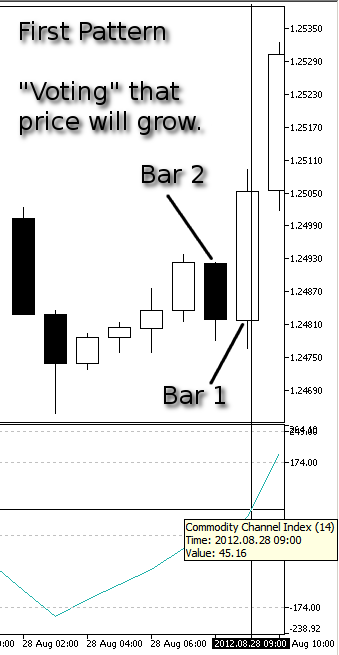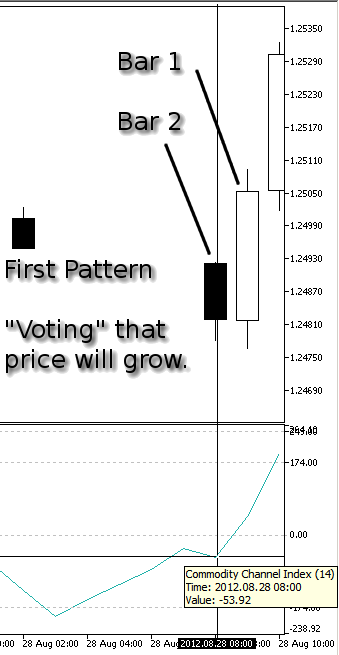• 图 7 显示对应于柱 1（当前柱的前一个柱）的 CCI 值。其值 -28.49 因此 < 0。
• 图 8 显示对应于柱 2（当前柱的前两个柱）的 CCI 值。其值 2.41 因此 > 0。
• 在 2 个柱内，CCI 指标的零线（值 0.00）被自上而下穿越。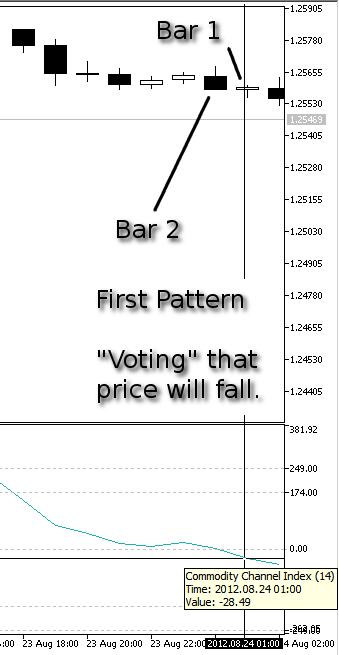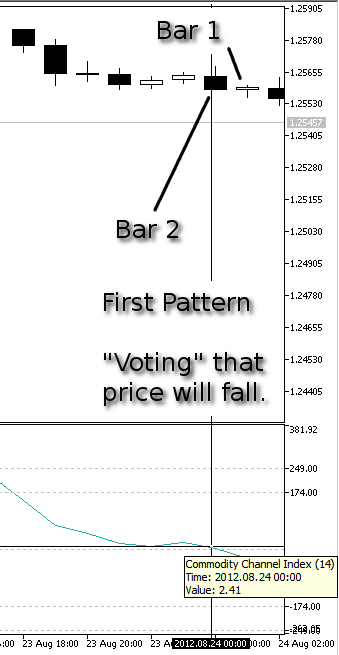### 第二个模式

• 图 9 显示对应于柱 1（当前柱的前一个柱）的 CCI 值。其值 119.06 因此 > 0。
• 图 10 显示对应于柱 2（当前柱的前两个柱）的 CCI 值。其值 -20.38 因此 < 0。
• 图 11 显示对应于柱 3（当前柱的前三个柱）的 CCI 值。其值 116.85 因此再次 > 0。
• CCI 指标的零线（值 0.00）被自上而下穿越。在 3 个柱内，CCI 指标线返回零线上方并在零线附近反弹。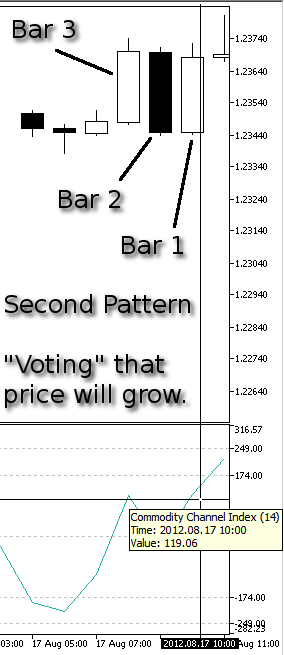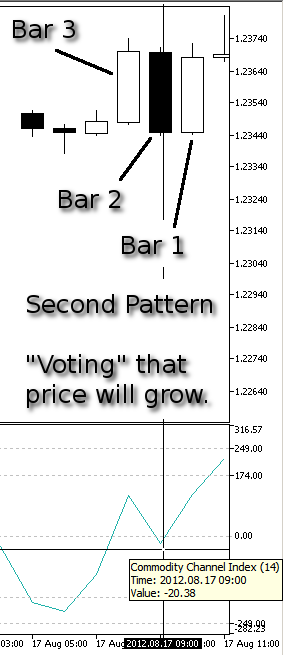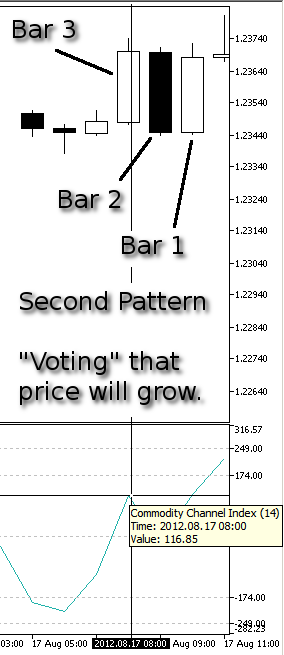• 图 12 显示对应于柱 1（当前柱的前一个柱）的 CCI 值。其值 -58.72 因此 < 0。
• 图 13 显示对应于柱 2（当前柱的前两个柱）的 CCI 值。其值 57.65 因此 > 0。
• 图 14 显示对应于柱 3（当前柱的前三个柱）的 CCI 值。其值 -85.54 因此再次 < 0。
• CCI 指标的零线（值 0.00）被自下而上穿越。在 3 个柱内，CCI 指标线返回零线下方并在零线附近反弹。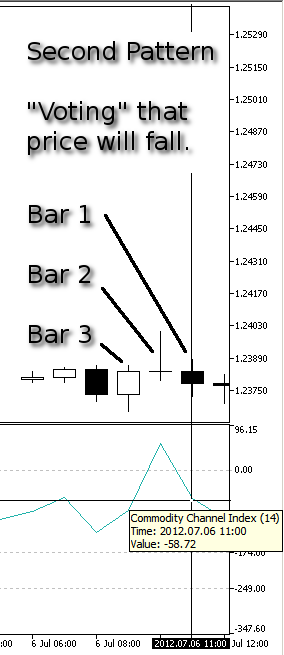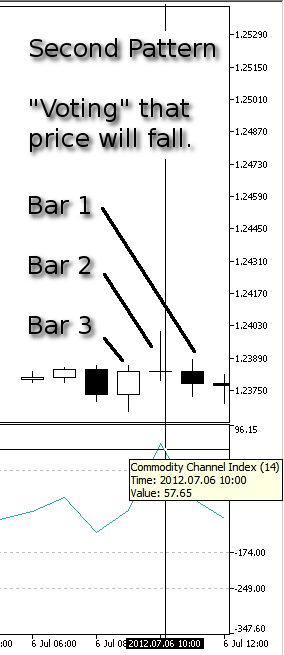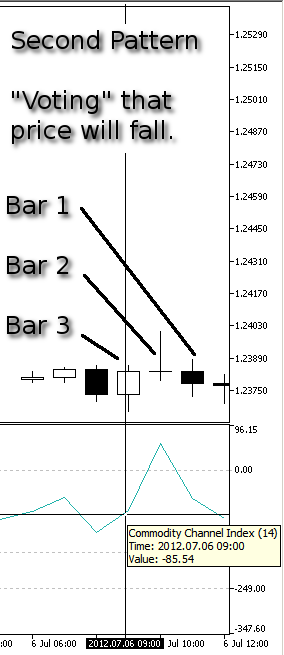### 实现模式

```//+------------------------------------------------------------------+
//| "Voting" that price will grow.                                   |
//| INPUT:  no.                                                      |
//| OUTPUT: number of "votes" that price will grow.                  |
//| REMARK: no.                                                      |
//+------------------------------------------------------------------+
int CSignalCCIxx::LongCondition()
{
int result=0;
int idx   =StartIndex();
//---
if(Diff(idx)>0.0)
{
//--- the oscillator is directed upwards confirming the possibility of price growth
if(IS_PATTERN_USAGE(0)) result=m_pattern_0;      // "confirming" signal number 0
//--- if the model 1 is used, search for a reverse of the oscillator upwards behind the level of overselling
if(IS_PATTERN_USAGE(1) && Diff(idx+1)<0.0 && CCIxx(idx+1)<-100.0)
result=m_pattern_1;      // signal number 1
//--- if the model 2 or 3 is used, perform the extended analysis of the oscillator state
if(IS_PATTERN_USAGE(2) || IS_PATTERN_USAGE(3))
{
ExtState(idx);
//--- if the model 2 is used, search for the "divergence" signal
if(IS_PATTERN_USAGE(2) && CompareMaps(1,1))      // 00000001b
result=m_pattern_2;   // signal number 2
//--- if the model 3 is used, search for the "double divergence" signal
if(IS_PATTERN_USAGE(3) && CompareMaps(0x11,2))   // 00010001b
return(m_pattern_3);  // signal number 3
}
// if the model 4 is used, look for crossing of the zero line
if(IS_PATTERN_USAGE(4) && CCIxx(idx+1)>0.0 && CCIxx(idx+2)<0.0)
result=m_pattern_4;      // signal number 4
// if the model 5 is used, look for the bouncing around the zero line
if(IS_PATTERN_USAGE(5) && CCIxx(idx+1)>0.0 && CCIxx(idx+2)<0.0 && CCIxx(idx+3)>0.0)
result=m_pattern_5;      // signal number 5
}
//--- return the result
return(result);
}```

```//+------------------------------------------------------------------+
//| "Voting" that price will fall.                                   |
//| INPUT:  no.                                                      |
//| OUTPUT: number of "votes" that price will fall.                  |
//| REMARK: no.                                                      |
//+------------------------------------------------------------------+
int CSignalCCIxx::ShortCondition()
{
int result=0;
int idx   =StartIndex();
//---
if(Diff(idx)<0.0)
{
//--- the oscillator is directed downwards confirming the possibility of falling of price
if(IS_PATTERN_USAGE(0)) result=m_pattern_0;      // "confirming" signal number 0
//--- if the model 1 is used, search for a reverse of the oscillator downwards behind the level of overbuying
if(IS_PATTERN_USAGE(1) && Diff(idx+1)>0.0 && CCIxx(idx+1)>100.0)
result=m_pattern_1;      // signal number 1
//--- if the model 2 or 3 is used, perform the extended analysis of the oscillator state
if(IS_PATTERN_USAGE(2) || IS_PATTERN_USAGE(3))
{
ExtState(idx);
//--- if the model 2 is used, search for the "divergence" signal
if(IS_PATTERN_USAGE(2) && CompareMaps(1,1))      // 00000001b
result=m_pattern_2;   // signal number 2
//--- if the model 3 is used, search for the "double divergence" signal
if(IS_PATTERN_USAGE(3) && CompareMaps(0x11,2))   // 00010001b
return(m_pattern_3);  // signal number 3
}
if(IS_PATTERN_USAGE(4) && CCIxx(idx+1)<0.0 && CCIxx(idx+2)>0.0)
result=m_pattern_4;      // signal number 4
if(IS_PATTERN_USAGE(5) && CCIxx(idx+1)<0.0 && CCIxx(idx+2)>0.0 && CCIxx(idx+3)<0.0)
result=m_pattern_5;      // signal number 5
}
//--- return the result
return(result);
}```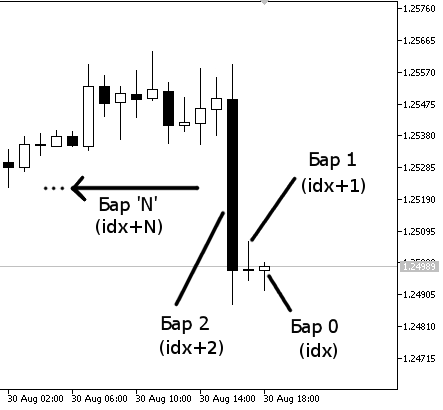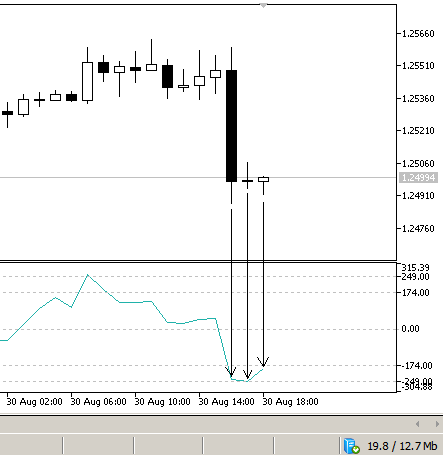`CCIxx(idx+1)>0.0 && CCIxx(idx+2)<0.0`

```CCI Indicator value (one bar before, named idx+1) is above the zero line of CCI indicator
AND
CCI Indicator value (two bars before, named idx+2) is below the zero line of CCI indicator
```

“价格将上涨”或“价格将下跌”的条件已用这种方式编写和加入“模式”中，并且完全允许创建更复杂的条件。在最终测试前，我们来看一看建仓/平仓的机制。

```//--- inputs for main signal
input int                Signal_ThresholdOpen =40;          // Signal threshold value to open [0...100]
input int                Signal_ThresholdClose=60;          // Signal threshold value to close [0...100]```

Signal_ThresholdOpen 是建买入持仓或卖出持仓的值，Signal_ThresholdClose 是平先前建立的仓位的值。这些值在紧附于向导生成的 EA 的整个逻辑的简单但聪明的机制的背景下进行计算。

```//--- setting default "weights" of the market models
m_pattern_0 =80;          // model 0 "price is on the necessary side from the indicator"
m_pattern_1 =10;          // model 1 "price crossed the indicator with opposite direction"
m_pattern_2 =60;          // model 2 "price crossed the indicator with the same direction"
m_pattern_3 =60;          // model 3 "piercing"
```

```//--- setting default "weights" of the market models
m_pattern_0  =70;         // model 0 "the oscillator has required direction"
m_pattern_1  =100;        // model 1 "reverse behind the level of overbuying/overselling"
m_pattern_2  =90;         // model 2 "failed swing"
m_pattern_3  =80;         // model 3 "divergence of the oscillator and price"
m_pattern_4  =100;        // model 4 "double divergence of the oscillator and price"
m_pattern_5  =20;         // model 5 "head/shoulders"
```

```//--- setting default "weights" of the market models
m_pattern_0  =90;         // model 0 "the oscillator has required direction"
m_pattern_1  =60;         // model 1 "reverse behind the level of overbuying/overselling"
m_pattern_2  =100;        // model 3 "divergence of the oscillator and price"
m_pattern_3  =50;         // model 4 "double divergence of the oscillator and price"
m_pattern_4  =80;         // model 4 "our own first new pattern: "
m_pattern_5  =90;         // model 5 "our own second new pattern: "```

```// if the model 4 is used, look for crossing of the zero line
if(IS_PATTERN_USAGE(4) && CCIxx(idx+1)>0.0 && CCIxx(idx+2)<0.0)
result=m_pattern_4;      // signal number 4 ```

• 信号 4 (m_pattern_4) 是条件为真的唯一信号（已激活信号）它达到 Signal_ThresholdOpen 的目标（其值乘以一个系数后达到并超过 Signal_ThresholdOpen 值）

• 信号 4 达到 Signal_ThresholdOpen 的目标，同时与 CCIxx 策略的其他对应信号竞争（CCIxx 策略的“投票价格将下跌”信号/模式），与其他指标（RSI 信号和 MA 信号）的相反方向（在这种情况下，相反方向是卖出持仓方向，因为我们正在分析的是关于“投票价格将上涨”的 m_pattern_4）的所有其他信号竞争。

• 在“价格上涨”条件下为 80
• 在“价格下跌”条件下为 -80

```//--- setting default "weights" of the market models
m_pattern_0 =0;          // model 0 "price is on the necessary side from the indicator"
m_pattern_1 =0;          // model 1 "price crossed the indicator with opposite direction"
m_pattern_2 =0;          // model 2 "price crossed the indicator with the same direction"
m_pattern_3 =0;          // model 3 "piercing"
```

```//--- setting default "weights" of the market models
m_pattern_0  =0;         // model 0 "the oscillator has required direction"
m_pattern_1  =0;        // model 1 "reverse behind the level of overbuying/overselling"
m_pattern_2  =0;        // model 2 "failed swing"
m_pattern_3  =0;        // model 3 "divergence of the oscillator and price"
m_pattern_4  =0;        // model 4 "double divergence of the oscillator and price"
m_pattern_5  =0;        // model 5 "head/shoulders"```

```//--- setting default "weights" of the market models
m_pattern_0  =0;        // model 0 "the oscillator has required direction"
m_pattern_1  =0;        // model 1 "reverse behind the level of overbuying/overselling"
m_pattern_2  =0;        // model 3 "divergence of the oscillator and price"
m_pattern_3  =0;        // model 4 "double divergence of the oscillator and price"
m_pattern_4  =80;       // model 4 "our own first new pattern: "
m_pattern_5  =0;        // model 5 "our own second new pattern: "```

```input int                Signal_CCIxx_PeriodCCI =8;            // Commodity Channel Index(8,...) Period of calculation
input ENUM_APPLIED_PRICE Signal_CCIxx_Applied   =PRICE_CLOSE;  // Commodity Channel Index(8,...) Prices series
input double             Signal_CCIxx_Weight    =0.8;          // Commodity Channel Index(8,...) Weight [0...1.0]```

```0.8 (Signal_CCIxx_Weight input parameter)
*
80 (m_pattern_4's weight value)
= 64 is the signal strength for the "voting that price will grow"```

```0.8 (Signal_CCIxx_Weight input parameter)
*
-80 (m_pattern_4's weight value)
= -64 = the negative value is considered for short positions```

-64 --> 64（绝对值）是“判断价格将下跌”的信号强度。信号强度始终以绝对值表示，同时在卖出持仓值前冠以减号，在买入持仓值前冠以加号。

```0.4 (the Signal_CCIxx_Weight)
*
80(m_pattern_4)
= 32 (strength of "long signal")
```

• 来自 SignalCCIxx.mqh 文件的 m_pattern_4（牛市）m_pattern_4（熊市），值分别为 80-80
• 来自 SignalRSI.mqh 文件的 m_pattern_1（牛市）m_pattern_1（熊市），值分别为 100-100
```m_pattern_4 Bullish --> 0.8 * 80 = 64
m_pattern_2 Bullish --> 0.7 * 100 = 70
```
```m_pattern_4 Bearish --> 0.8 * (-80) = -64
m_pattern_2 Bearish --> 0.7 * (-100) = -70
```

```A) m_pattern_4 Bullish + m_pattern_2 Bullish = {[0.8 * (80)] + [0.7 * (100)]}/2 = [64 + (70)]/2 = 134/2 = 67
B) m_pattern_4 Bullish + m_pattern_2 Bearish = {[0.8 * (80)] + [0.7 * (-100)]}/2 = [64 + (-70)]/2 = -6/2 = -3
C) m_pattern_4 Bearish + m_pattern_2 Bullish = {[0.8 * (-80)] + [0.7 * (100)]}/2 = [(-64) + 70]/2 = 6/2 = 3
D) m_pattern_4 Bearish + m_pattern_2 Bearish = {[0.8 * (-80)] + [0.7 * (-100)]}/2 = [(-64) + (-70)]/2 = -134/2 = -67
```

### 仓位细节

• 正常交易。仓位建立然后平仓。之后，仓位没有立即重新建立。
• 仓位反向。仓位建立然后平仓，接下来以反方向再次建立。

`Open_long >= Signal_ThresholdOpen`

 我们收到一个卖出信号，因此买入持仓将反向，如果：`Open_short > Signal_ThresholdClose AND Open_short > Signal_ThresholdOpen` 我们收到一个卖出信号，因此买入持仓将平仓，如果：`Open_short > Signal_ThresholdClose AND Open_short < Signal_ThresholdOpen`

 我们收到一个卖出信号，因此买入持仓将反向，如果：`Open_short > Signal_ThresholdClose OR Open_short > Signal_ThresholdOpen` 我们收到一个卖出信号，因此买入持仓将平仓，如果：`Open_short > Signal_ThresholdClose OR Open_short < Signal_ThresholdOpen`

`Open_short >= Signal_ThresholdOpen.`
Signal_ThresholdClose <= Signal_ThresholdOpen
 我们收到一个买入信号，因此卖出持仓将反向，如果：`Open_long > Signal_ThresholdClose AND Open_long > Signal_ThresholdOpen` 我们收到一个买入信号，因此卖出持仓将平仓，如果：`Open_long > Signal_ThresholdClose AND Open_long < Signal_ThresholdOpen`

 我们收到一个买入信号，因此卖出持仓将反向，如果：`Open_long > Signal_ThresholdClose OR Open_long > Signal_ThresholdOpen` 我们收到一个买入信号，因此卖出持仓将平仓，如果：`Open_long > Signal_ThresholdClose OR Open_long < Signal_ThresholdOpen`

### 价格水平和信号过期

`input double             Signal_PriceLevel    =0.0;         // Price level to execute a deal`

• Signal_PriceLevel 决定是将买入持仓信号作为买入止损还是买入限价处理，或将卖出持仓信号作为卖出止损或卖出限价处理。有关止损订单和限价订单的详细内容，请参见相应的 MetaTrader 5 帮助部分

• 分配给输入变量 Signal_PriceLevel 的负值始终表示止损订单（买入或卖出）。

• 分配给输入变量 Signal_PriceLevel 的正值始终表示限价订单（买入或卖出）。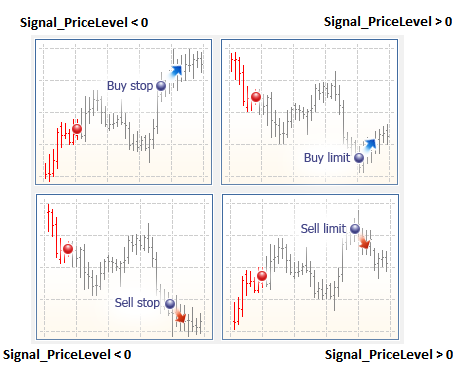EURUSD - 买入持仓

Signal_PriceLevel = -70（负 70）
，因此当激活建仓信号时（例如，当前价格 = 1.2500），
EA 将下达由 1.2500 + 70 = 1.2570

Signal_PriceLevel = 60（正 60）
，因此当激活建仓信号时（例如，当前价格 = 1.2500），
EA 将下达由 1.2500 - 60 = 1.2440

EURUSD - 卖出持仓

Signal_PriceLevel = -70（负 70）
，因此当激活建仓信号时（例如，当前价格 = 1.2500），
EA 将下达由 1.2500 - 70 = 1.2430

Signal_PriceLevel = 60（正 60）
，因此当激活建仓信号时（例如，当前价格 = 1.2500），
EA 将下达由 1.2500 + 60 = 1.2560

`input int                Signal_Expiration    =4;           // Expiration of pending orders (in bars)`

### 流程图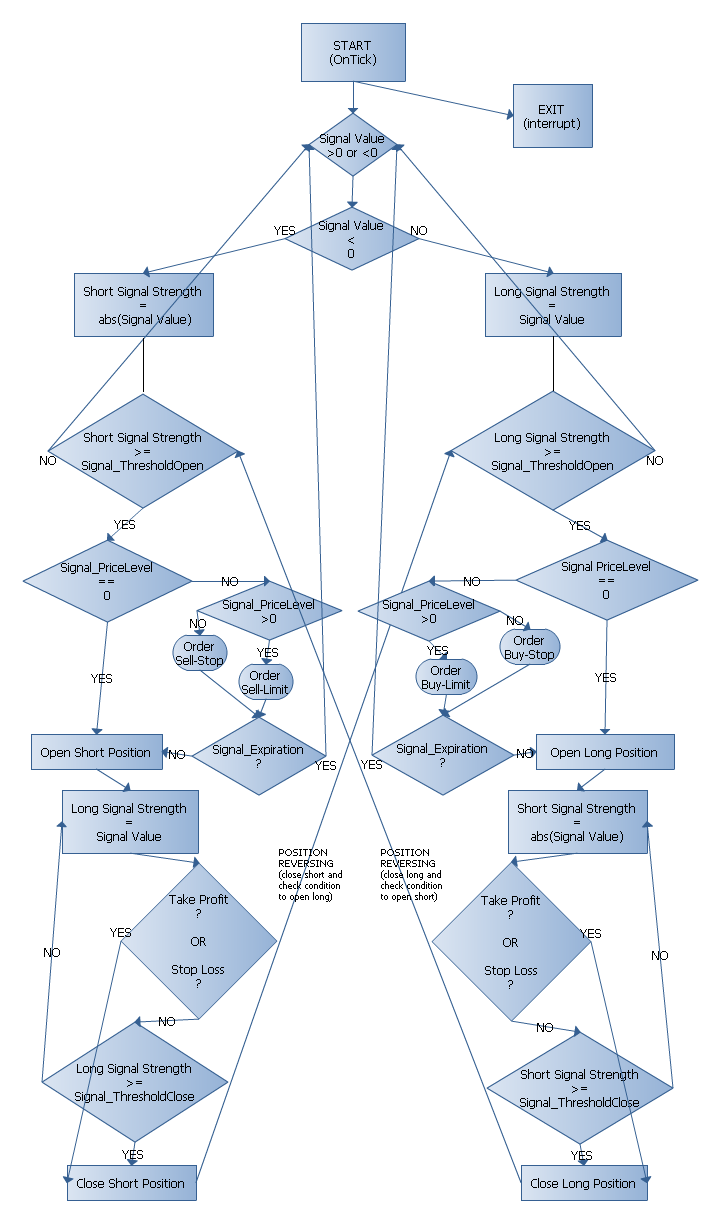### 策略测试程序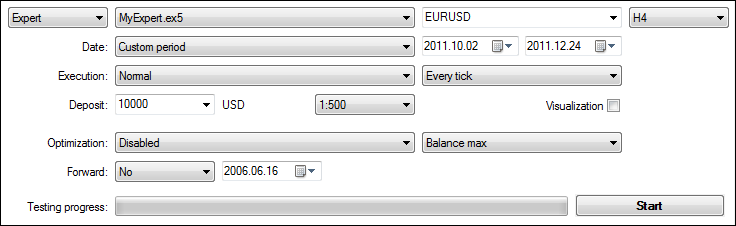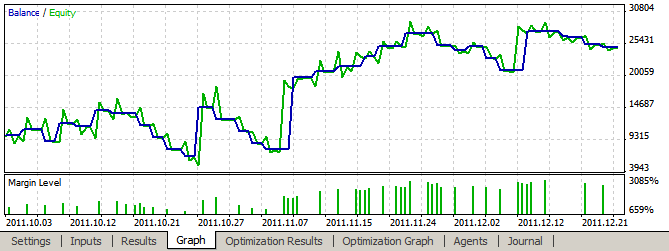### 总结

myexpert.mq5 (8.57 KB)
signalccixx.mqh (21.02 KB)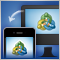MetaTrader 移动终端中的 MetaQuotes ID

Android 和 iOS 设备为我们提供了许多功能，有一些甚至连我们自己都不知道。这些功能的其中之一就是推送通知，这允许我们接收个人消息，而不会拘于我们的电话号码或移动网络运营商。MetaTrader 移动终端已经能够从您的自动交易接收这样的消息。您只需要知道设备的 MetaQuotes ID。超过 9 000 000 台移动终端已经收到它。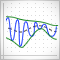经验模态分解法介绍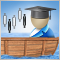统计套利交易策略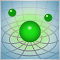神经网络：从理论到实践